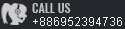Technical DetailsCOMPARISON OF SHOCK ABSORBING OF DASH POTS, PU RUBBERS, SPRINGS AND CEC SHOCK ABSORBERS

FORCE-STROKE TESTNG MACHINE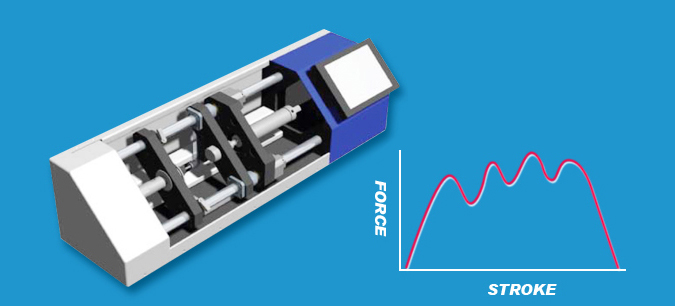When shock absorber was affected by the force from outside, the main function of this testing machine is testing the relative curve between the pressure of inner tube undertake and the stroke of the shock absorber. That means through this curve, we can judge the efficiency of the shock absorber absorb the kinetic energy by the force it was affected.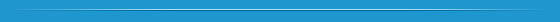The springs and polyurethane rubbers are widespread to use in earlier period, but due to provide non-linear deceleration and to result in strong resistance, all the kinetic energy of moving objects is not absorption and produce counter pressure, this is in low efficiency. If linear deceleration is necessary for a moving object, CEC Shock Absorbers is your best choice.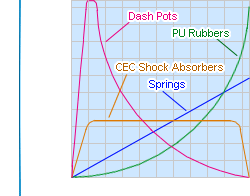Examples of using CEC shock absorbers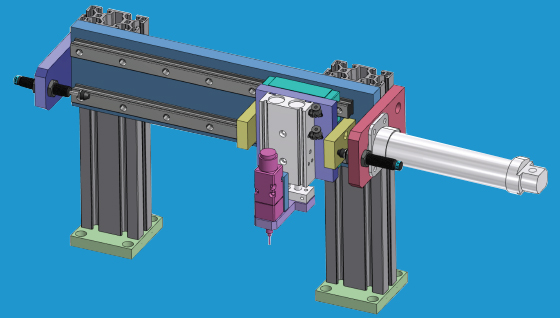OPERATING PRINCIPAL OF SHOCK ABSORBERS Top

CEC Shock Absorbersâ main structure to combine with body, rod, bearing, inner tube, piston, fluid, spring. On impact the piston rod moves into the shock absorbers and the hydraulic fluid is pushed into the accumulator to produce resistant force, the pressure in the inner tube remains constant throughout the entire impact stroke. CEC Shock Absorbers providing a linear decelerationand brings the impacting object to stop smoothly and quietly. At the end of the impact stroke, the return spring pushes the piston to the original position for next cycle.ROD BEARING PISTON INNER TUBE BODY
 SELECTION OF HYRAULIC SHOCK ABSORBERS Top

â²Three major factors to be consideredï¼
 1 How much energy to be dissipated each stroke? 2 How much energy to be dissipated per hour? 3 How much is your effective weight of your application?
After the three factors are calculated, go to the quick list to find the suitable shock absorbers.Note:
 1 Shock absorbers series allow approximate 1 mm fixed stop before the end of the stroke, not to be driven into the final position under full load. 2 Reuse is prohibited after disassembling; paint on the rod and threaded body is not allowed. 3 Stop collar protects shock absorberâs piston from bottoming out, Stop collar can be used in adjust the distance in stroke. Be sure the fixed board of the shock absorbers is strong enough. 4 To assembling, the off-center angle do not exceed 2Â°.

 SYMBOLS AND FORMULAS Top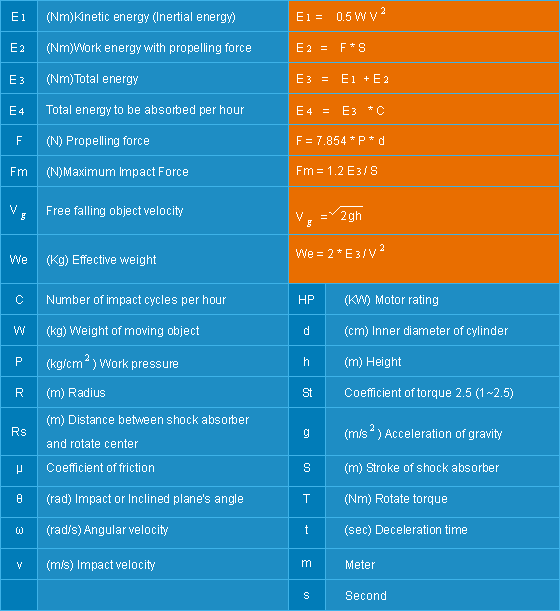APPLICATION EXAMPLES Top

ex. 1 Horizontal Impact without Propelling Force(Application) W = 20 kg V = 1 m / s C = 1000 / Hr(Formulas and Calculation) E1 = 0.5 x W x V2 E2 = 0 E3 = E1 + E2 E4 = E3 x C We = WE1 = 0.5 x 20 x 12 = 10 Nm E2 = 0 E3 = 10 + 0 = 10 Nm / C E4 = 10 x 1000 = 10000 Nm / Hr We = 20 kg Model SC1415-1 is adequate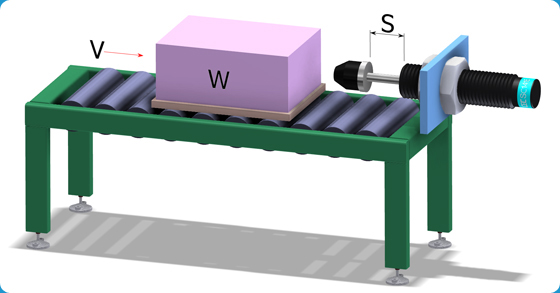ex. 2 Horizontal Impact with Conveyor Driving(Application) W = 10 kg V = 1 m / s C = 600 / Hr S = 0.01 m Î¼ = 0.25(Formulas and Calculation) E1 = 0.5 x W x V2 E2 = W x Î¼ x g x S E3 = E1 + E2 E4 = E3 x C We = 2 x E3 / V2E1 = 0.5 x 10 x 12 = 5 Nm E2 = 10 x 0.25 x 9.81 x 0.01 = 0.25 Nm E3 = 5 + 0.25 = 5.25 Nm / C E4 = 5.25 x 600 = 3,150 Nm / Hr We = 2 x 5.25 / 12 = 10.5 kg Model SC1210-2 is adequate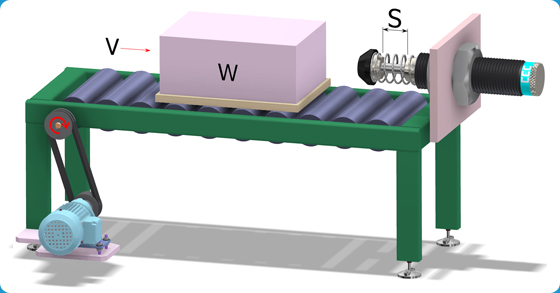ex. 3 Horizontal Impact with Propelling Force(Application) W = 50 kg V = 1 m / s F = 1000 N C = 500 / Hr S = 0.04 m(Formulas and Calculation) E1 = 0.5 x W x V2 E2 = F x S E3 = E1 + E2 E4 = E3 x C We = 2 x E3 / V2E1 = 0.5 x 50 x 12 = 25 Nm E2 = 1000 x 0.04 = 40 Nm E3 = 25 + 40 = 65 Nm / C E4 = 65 x 500 = 32500 Nm / Hr We = 2 x 65 / 12 = 130 kg Model FC2540 is adequate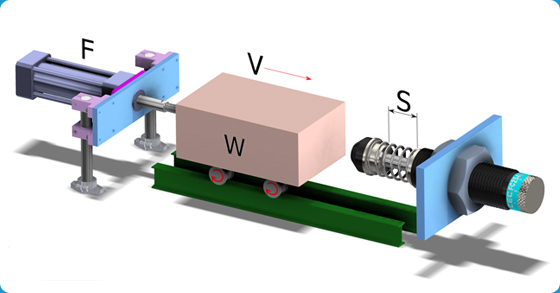ex. 4 Vertical Impact with Force from Top to Bottom(Application) W = 100 kg V = 1 m / s F = 1200 N C = 400 / Hr S = 0.025 m(Formulas and Calculation) E1 = 0.5 x W x V2 E2 = ( F + W x g ) x s E3 = E1 + E2 E4 = E3 x C We = 2 x E3 / V2E1 = 0.5 x 100 x 12 = 50 Nm E2 = ( 1200 + 100 x 9.81 ) x 0.25= 54.5 Nm E3 = 50 + 54.5 = 104.5 Nm / C E4 = 104.5 x 400 = 41800 Nm / Hr We = 2 x 104.5 / 12 = 209 kg Model FC3625 is adequate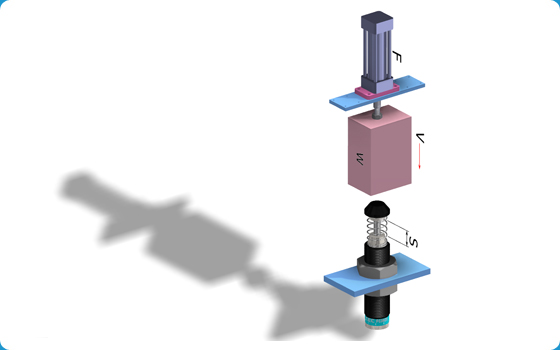ex. 5 Vertical Impact with Force from Bottom to Top(Application) W = 200 kg V = 0.5 m / s F = 3000 N C = 500 / Hr S = 0.05 m(Formulas and Calculation) E1 = 0.5 x W x V2 E2 = ( F - W x g ) x s E3 = E1 + E2 E4 = E3 x C We = 2 x E3 / V2E1 = 0.5 x 200 x 0.52 = 25 Nm E2 = ( 3000 - 200 x 9.81 ) x 0.05 = 51.9 Nm E3 = 25 + 51.9 = 76.9 Nm / C E4 = 76.9 x 500 = 38450 Nm / Hr We = 2 x 76.9 / 0.52 = 615.2 kg Model FC3650 is adequate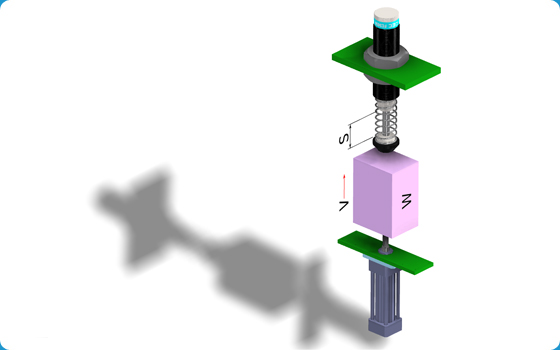ex. 6 Horizontal Impact with Motor Driving(Application) W = 50 kg V = 1.5 m / s ST = 2.5 HP = 2 KW C = 100 / Hr S = 0.06 m(Formulas and Calculation) E1 = 0.5 x W x V2 E2 = 1000 x HP x ST x S / V E3 = E1 + E2 E4 = E3 x C We = 2 x E3 / V2E1 = 0.5 x 50 x 1.52 = 56.25 Nm E2 = 1000 x 2 x 2.5 x 0.06 / 1.5 = 200 Nm E3 = 56.25 + 200 = 256.25 Nm / C E4 = 256.25 x 100 = 25625 Nm / Hr We = 2 x 256.25 / 1.52 = 227 kg Model SC3660-2 is adequate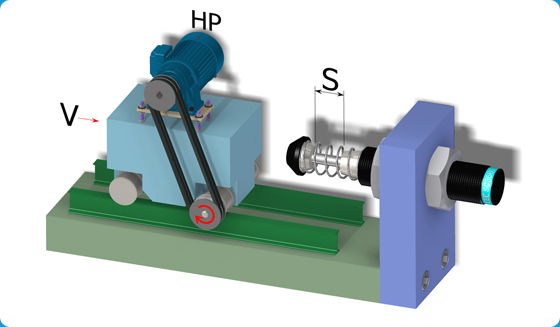ex. 7 Free Fall Impact(Application) W = 300 kg h = 0.5 m C = 300 / Hr S = 0.08 m(Formulas and Calculation) E1 = W x g x h E2 = W x g x s E3 = E1 + E2 E4 = E3 x C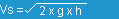We = 2 x E3 / V2E1 = 30 x 9.81 x 0.5= 147 Nm E2 = 30 x 9.81 x 0.08 = 23.5 Nm E3 = 147 + 23.5 = 170.5 Nm / C E4 = 170.5 x 300 = 51150 Nm / Hr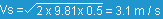We = 2 x 170.5 / 3.12 = 35.5 kg Model SC2580-1 is adequate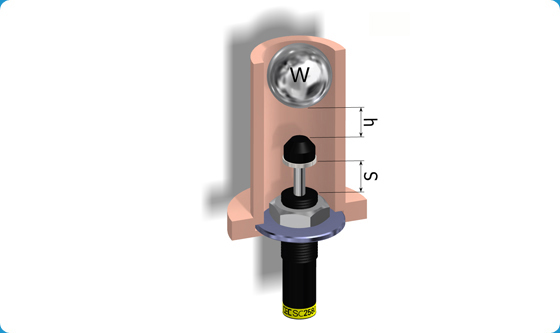ex. 8 Free Moving Load Down an Inclined Plane(Application) W = 30 kg L = 1 Î¸ = 30Â° S = 0.04 C = 250 / Hr(Formulas and Calculation)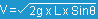E1 = 0.5 x W x V2 E2 = W x S x SinÎ¸ E3 = E1 + E2 E4 = E3 x C We = 2 x E3 / V2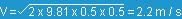E1 = 0.5 x 30 x 2.22 = 72.6 Nm E2 = 30 x 0.04 x 9.81 x 0.5 = 5.9 Nm E3 = 72.6 + 5.9 = 78.5 Nm / C E4 = 78.5 x 250 = 19625 Nm / Hr We = 2 x 78.5 / 2.22 = 32 kg Model SC2540-1 is adequate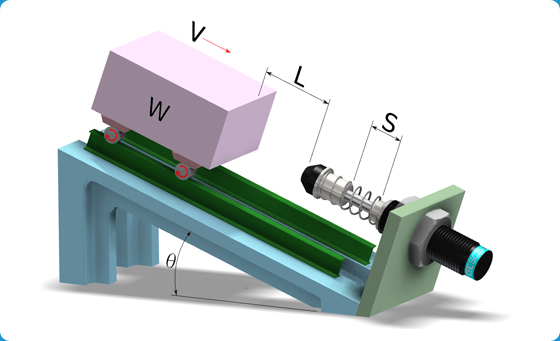ex. 9 Rorary with Propelling Force(Application) W = 100 kg V = 1.1 m / s T = 2000 Nm S = 0.06 m RT = 1.25 m RS = 0.8 m C = 100 / Hr(Formulas and Calculation) E1 = 0.25 x W x V2 E2 = ( T x S ) / RS E3 = E1 + E2 E4 = E3 x C Vs=( VT x RS ) / RT We = 2 x E3 / Vs2E1 = 0.25 x 100 x 1.12 = 30.3 Nm E2 = 2000 x 0.06 / 0.8 = 150 Nm E3 = 30.3 + 150 = 180.3 Nm / C E4 = 180.3 x 100 = 18030 Nm / Hr Vs = 1.1 x 0.8 / 1.25 = 0.7 m / s We = 2 x 180.3 / 0.72 = 736 kg Model SC3660-3 is adequate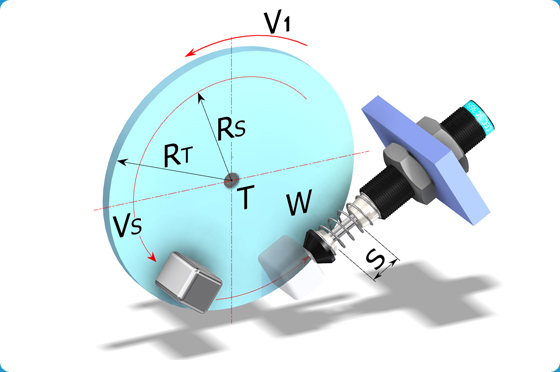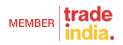CEC YUH BAW CO., LTD. All Rights Reserved.(Terms of Use)Developed and Managed by Infocom Network Ltd.